Courses

NCERT Solution (Ex- 3.3) - Chapter 3: Understanding Quadrilaterals, Maths, Class 8 Notes - Class 8

Class 8: NCERT Solution (Ex- 3.3) - Chapter 3: Understanding Quadrilaterals, Maths, Class 8 Notes - Class 8

The document NCERT Solution (Ex- 3.3) - Chapter 3: Understanding Quadrilaterals, Maths, Class 8 Notes - Class 8 is a part of Class 8 category.
All you need of Class 8 at this link: Class 8

Exercise 3.3

Question 1:
Given a parallelogram ABCD. Complete each statement along with the definition or property used.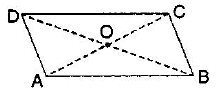(ii)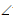DCB = ____________________
(iii) OC = ____________________
(iv) mDAB + mCDA = ____________________

(i) AD = BC   [Since opposite sides of a parallelogram are equal]
(ii)DCB =DAB  [Since opposite angles of a parallelogram are equal]
(iii) OC = OA    [Since diagonals of a parallelogram bisect each other]
(iv) mDAB + mCDA = 180o   [Adjacent angles in a parallelogram are supplementary]

Question 2:
Consider the following parallelograms. Find the values of the unknowns x, y, ∠.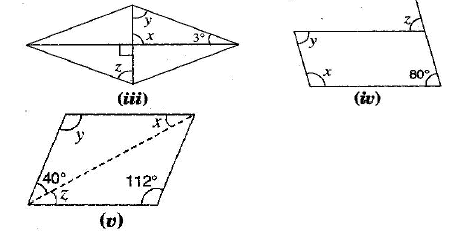(i)B +C = 180o [Adjacent angles in a parallelogram are supplementary]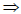100o+ x =180ox =180o-100o = 80o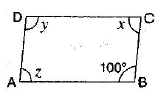and ∠= x = 80o    [Since opposite angles of a parallelogram are equal]
also y =100o     [Since opposite angles of a parallelogram are equal]
(ii) x +50o =180o    [Adjacent angles in a ||gm are supplementary]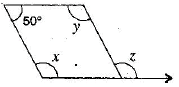x =180o- 50o =130o∠= x =130o [Corresponding angles]

(iii) x = 90o    [Vertically opposite angles]y + x +30o =180o  [Angle sum property of a triangle]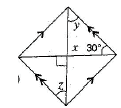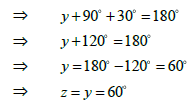[Alternate angles]

(iv) ∠= 80o   Corresponding angles]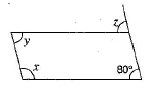x +80o = 180o  [Adjacent angles in a ||gm are supplementary]x =180o- 80o = 100o

and y = 80o    [Opposite angles are equal in a ||gm]

(v) y =112o [Opposite angles are equal in a ||gm]40o + y + x =180o [Angle sum property of a triangle]40o+112o+ x =180o152o+ x =180o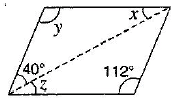x =180o- 152o = 28o
and ∠= x = 28o [Alternate angles]

Question 3:
Can a quadrilateral ABCD be a parallelogram, if:
(i)D +B = 180o
(ii) AB = DC = 8 cm, AD = 4 cm and BC = 4.4 cm?
(iii)A = 70o andC = 65o

(i)D +B = 180o
It can be, but here, it needs not to be.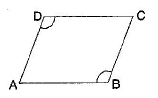(ii) No, in this case because one pair of opposite sides are equal and another pair of opposite sides are unequal. So, it is not a parallelogram.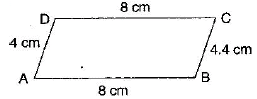(iii) No.A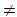C.
Since opposite angles are equal in parallelogram and here opposite angles are not equal in quadrilateral ABCD. Therefore it is not a parallelogram.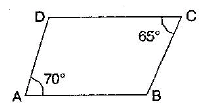Question 4:
Draw a rough figure of a quadrilateral that is not a parallelogram but has exactly two opposite angles of equal measures.

ABCD is a quadrilateral in which anglesA =C = 110o.

Therefore, it could be a kite.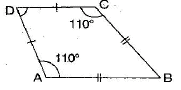Question 5:
The measure of two adjacent angles of a parallelogram are in the ratio 3:2. Find the measure of each of the angles of the parallelogram.
:
Let two adjacent angles be 3x and 2 . x
Since the adjacent angles in a parallelogram are supplementary.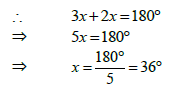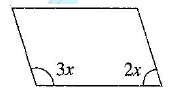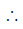One angle = 3x = 3x36o =108o
and another angle = 2x = 2x36o = 72o

Question 6:
Two adjacent angles of a parallelogram have equal measure. Find the measure of the angles of the parallelogram.

Let each adjacent angle be x.
Since the adjacent angles in a parallelogram are supplementary.x + x =180o2x =180o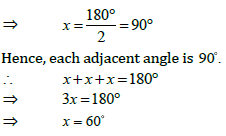Question 7:
The adjacent figure HOPW is a parallelogram. Find the angle measures x, y and ∠. State the properties you use to find them.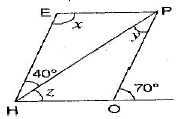HereHOP + 70o =180o[Angles of linear pair]HOP = 180o- 70o = 110o
andE =HOP [Opposite angles of a ||gm are equal]x =110o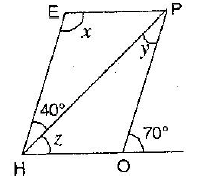∠ PHE = ∠HPO [Alternate angles]

∴ y = 400

Now ∠ EHO = ∠O = 70° [Corresponding angles]

=> 40° ∠ = 70°

=> ∠ = 70°- 40° = 30°

Hence,

x = 110° , y = 40° and ∠ =30°

Question 8:

The following figures GUNS and RUNS are parallelograms. Find x and y. (Lengths are in cm)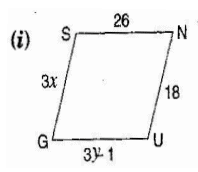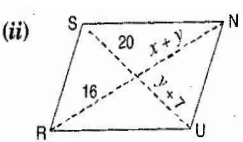(i) In parallelogram GUNS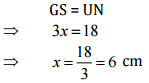[ Opposite sides of parallelogram are equal]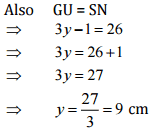[ Opposite sides of parallelogram are equal]

Hence, x = 6cm, and y = 9 cm

(ii)  In parallelogram RUNS

y + 7 = 20 [ Diagonals of ||gm bisects each other]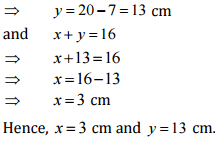Question 9:

In the figure, both RISK and CLUE are parallelograms. Find the value of x.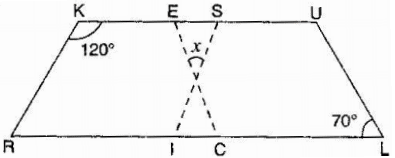In Parallelogram RISK,

∠RIS = ∠K = 1200

[Opposite angles of A ||gm bisects each other]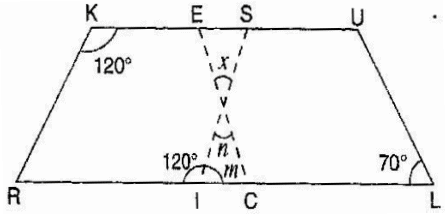∠m +120° = 180° [Linear pair]

=> ∠m = 180°-120° = 60°

and

=> ∠ ECI = ∠ L = 70° [Corresponding angles]

=> m + n + ∠ECl = 180° [Angle sum property of a triangle]

60°+n+ 70° = 180°

=> 130°+n = 180°

=> n= 180°-130° = 50°

also x = n = 50° [Vertically opposite angles]

Question 10:

Explain how this figure is a trape∠ium. Which is its two sides are parallel?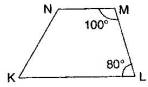Here, M + L = 100° + 80 ° = 180° [Sum of interior opposite angles is 180°]

NM and KL are parallel.

Hence, KLMN is a trapezium

Question 11: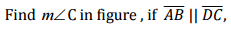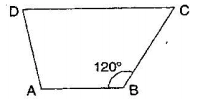Here, ∠B + ∠C = 1800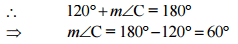Question 12:

Find the measure of ∠P and ∠S if SP || RQ in given figure.

(If you find mR is there more than one method to find m∠P)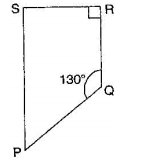Here, ∠P + ∠Q = 180° [Sum of co-interior angles is 180° ]

=> ∠P + 130° = 180°

∠P = 180°-130°

=> ∠P = 50°

∴∠R = 900

∠S + 90° = 180° [Given]

∠S = 180°-90°

=> ∠s = 90°

Yes, one more method is there to find ∠P.

∠S+∠R+∠Q + ∠P = 360° [Angle sum property of quadrilateral]

90°+90° +130°+∠P = 360°

=> 310° + ∠P = 360°

=> ∠P = 360°-310°

=> ∠P = 50°

The document NCERT Solution (Ex- 3.3) - Chapter 3: Understanding Quadrilaterals, Maths, Class 8 Notes - Class 8 is a part of Class 8 category.
All you need of Class 8 at this link: Class 8Use Code STAYHOME200 and get INR 200 additional OFF Use Coupon Code

Top Courses for Class 8Top Courses for Class 8Track your progress, build streaks, highlight & save important lessons and more!

,

,

,

,

,

,

,

,

,

,

,

,

,

,

,

,

,

,

,

,

,

,

,

,

,

,

,

;